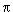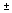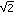# Number Sets, Infinity, and Zero Part A: Number Sets (35 minutes)

In This Part: Relating Number Sets
We will continue our focus on the number line and the relationships among the various types of numbers that make up the real number system. The following exercises will help you further understand the properties that hold true for each of the sets of numbers and the relationships among them.

As we saw in Session 1, the real number system is made up of many different sets. Some of these sets are quite large and contain other smaller sets. The integers, for example — made up of the whole numbers and their negatives — clearly contain the counting numbers (1, 2, 3, 4, …). But which sets contain which other sets, and how do they all relate to one another? Let’s explore.

Problem A1
Using the number line from Session 1 as a reference, draw a diagram that illustrates the relationships among the different sets of numbers that make up our number system — the real numbers plus imaginary and complex numbers. Include all of the sets we discussed in Session 1:

• Counting numbers (1, 2, 3, 4, …)
• Whole numbers (the counting numbers and 0)
• Integers (positive and negative whole numbers)
• Rational numbers (numbers that can be expressed as a ratio of two integers; when expressed in a decimal form, they will either terminate or repeat)
• Irrational numbers (numbers such asor e or square roots that can’t be expressed as a ratio of two integers; they can be expressed as infinite, non-repeating decimals)
• Transcendental numbers (numbers that cannot be the solution to a polynomial equation; e.g.,and e)
• Real numbers (all rational and irrational numbers; numbers that can be represented on a number line)
• Algebraic numbers are solutions to polynomial equations with rational coefficients (e.g., 1/2x3 – 3x2 +17x +5/8). They include all integers, rational numbers, and some irrational numbers (e.g.,, the solutions to x2 – 2 = 0). Algebraic numbers also include some complex numbers (e.g.,-1, the solutions to x2 + 1 = 0).
• Pure imaginary numbers (multiples of i, a number such that when you square it, you get -1; e.g., 5i, 99i)
• Complex numbers (numbers created by the addition of imaginary and real number elements; e.g., 1 + 5i; 3/2 + 19i)Use boxes or circles to represent each number set. Shapes that represent number sets should be placed within their larger set in the number system.

In This Part: Operations
Compare and contrast your diagram with the diagram below, which shows one way to illustrate the relationships among sets of numbers.Note 2

Problem A2
Which operations can we do within the following sets: counting numbers, whole numbers, integers, irrational numbers and rational numbers (i.e., for each set decide under which operations is it closed)?Select a set and try adding, subtracting, multiplying, or dividing random numbers from that set. What happens?

Problem A3
a.
Within each set, which operations require us to expand to a new set?
b. To go from one set to the next biggest, what new types of numbers do you need to include?Video Segment
In this video segment, Donna and Susan contemplate the relationships between different sets of numbers in the real number system. They discuss the operations and how different operations require them to expand the number sets they’re using. Watch this segment after you’ve completed Problems A1-A3.
You can find this segment on the session video approximately 4 minutes and 7 seconds after the Annenberg Media logo.

### Notes

Note 1
These diagrams are known as Venn diagrams. To learn more about Venn diagrams, go to Learning Math: Number and Operations, Session 6, and Learning Math: Geometry, Session 3, Part B: Game.

Note 2
The fact that all algebraic numbers lie within the complex numbers was proven by a German mathematician, Carl Friedrich Gauss, and is known as the Fundamental Theorem of Algebra.

### Solutions

Problem A1Problem A2
For each set, you can only do operations for which that set is closed:

• Counting Numbers: This set is closed only under addition and multiplication. In other words, we can solve all addition and multiplication problems, but not all subtraction and division problems are solvable.
• Whole Numbers: This set is closed only under addition and multiplication.
• Integers: This set is closed only under addition, subtraction, and multiplication.
• Rational Numbers: This set is closed under addition, subtraction, multiplication, and division (with the exception of division by 0).
• Irrational Numbers: This set is closed for none of the operations (e.g.,•= 2, a rational number).
• Real Numbers: This set is closed only under addition, subtraction, multiplication, and division (with the exception of division by 0).

Problem A3
a.
Operations under which a particular set is not closed require new sets of numbers:

• Counting Numbers: Subtraction requires 0 and negative integers; division requires rational numbers.
• Whole Numbers: Subtraction requires negative integers; division requires rational numbers.
• Integers: Division requires rational numbers.
• Rational Numbers: All four operations are okay here (with the exception of division by 0). However, solving problems with exponents would require us to expand from the rational numbers. For example, a problem like x2 = 3 can be solved using the real numbers, but not the rational numbers.
• Irrational Numbers: All operations require rational numbers.
• Real Numbers: All four operations are okay here (with the exception of division by 0).

b. To go from one set to the next requires new types of numbers:

• To go from counting numbers to whole numbers, we need the additive identity 0.
• To go from whole numbers to integers, we need the additive inverses — the opposites of the counting numbers.
• To go from integers to rational numbers, we need the multiplicative inverses of all non-zero counting numbers and their multiples. These are fractions with integer numerators and denominators, like 2/3 and -7/4.
• To go from rational numbers to real numbers, we need irrational numbers, such asand. Similarly, to go from irrational to real numbers, we need rational numbers.
• To go from real numbers to complex numbers, we need i (a number such that when squared it gives -1) and all its real multiples — the imaginary numbers. Adding any real number and any imaginary number then forms a complex number, for example, 2 + 3i and -2/3 + 2.718i.